# Doc:2.6/Manual/Materials/Nodes/Types/Convertor

< Doc:2.6‎ | Manual‎ | Materials‎ | Nodes‎ | Types

# Material Convertor Nodes

As the name implies, these nodes convert the colors in the material in some way.

## ColorRamp Node

The ColorRamp Node is used for mapping values to colors with the use of a gradient. It works exactly the same way as a Colorband for textures and materials, using the Factor value as a slider or index to the color ramp shown, and outputting a color value and an alpha value from the output sockets.

By default, the ColorRamp is added to the node map with two colors at opposite ends of the spectrum. A completely black black is on the left (Black as shown in the swatch with an Alpha value of 1.00) and a whitewash white is on the right.

To select a color, click on the thin vertical line/band within the colorband. The example picture shows the black color selected, as it is highlighted white. The settings for the color are shown above the colorband as (left to right): color swatch, Alpha setting, and interpolation type.

### Inputs

Fac
Factor. The degree of node's influence in node tree. The value can be provided by another node or set manually.

### Outputs

Color
Value of the color, combined by the node.
Alpha
Value of the alpha, combined by the node.

### Controls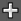Add a new mark to the center of the colorband with the default color (neutral gray).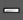Remove the currently selected mark from the colorband.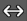Flip the colorband.

Interpolation
Various modes of interpolation between marker's values can be chosen in the Interpolation menu:
Ease
Cardinal
Cardinal.
Linear
Linear (default). A smooth, consistent transition between colors.
B-Spline
B-Spline.
Constant
Constant.

Colorband
Contain a gradient through a sequence of many colors (with alpha), each color acting across a certain position in the spectrum.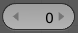The number of the active mark.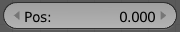Pos. The position of the active color mark in the colorband (range 0.0–1.0). The position of the color marks can also be changed by dragging them in the colorband.

Color Selector
Allows set color and alpha values for each marker.

See more details about node controls' functions here.

## RGB to BW Node

This node converts a color image to black-and-white.

### Inputs

Color
Input color value. Includes a color swatch, allowing you to select the color directly on the node.

### Outputs

Value
Black-and-white value of the input color, converted by the node.

## Math Node

This node performs the selected math operation on an image or buffer. All common math functions are supported. If only an image is fed to one Value socket, the math function will apply the other Value consistently to every pixel in producing the output Value. Select the math function by clicking the up-down selector where the "Add" selection is shown.

### Inputs

Value
Input value 1 (upper). The value can be provided by another node or set manually.
Value
Input value 2 (lower). The value can be provided by another node or set manually.

### Outputs

Value
Output value, converted by the node.

### Controls

Clamp
Clamps the result between 0 and 1.
Operation
Selector the math function for conversion.
Subtract
Subtract input 2 from input 1
Multiply
Multiply the two inputs
Divide
Divide input 1 by input 2
Sine
The sine of input 1 (degrees)
Cosine
The cosine of input 1 (degrees)
Tangent
The tangent of input 1 (degrees)
Arcsine
The arcsine (inverse sine) of input 1 (degrees)
Arccosine
The arccosine (inverse cosine) of input 1 (degrees)
Arctangent
The arctangent (inverse tangent) of input 1 (degrees)
Power
Input 1 to the power of input 2 (input1^input2)
Logarithm
Log base input 2 of input 1
Minimum
The minimum of input 1 and input 2
Maximum
The maximum of input 1 and input 2
Round
Rounds input 1 to the nearest integer
Less Than
Test if input 1 is less than input 2, returns 1 for true, 0 for false
Greater Than
Test if input 1 is greater than input 2, returns 1 for true, 0 for false
Modulo
Division of input 1 by input 2 with remainder.
Absolute
Always return non-negative value from any operation input 2 between input 1.

## Vector Math Node

This node performs the selected math operation on vectors. Select the math function by clicking the up-down selector where the "Add" selection is shown.

### Inputs

Vector
Input vector 1 (upper). The value can be provided by another node or set manually.
Vector
Input vector 2 (lower). The value can be provided by another node or set manually.

### Outputs

Vector
Output vector, converted by the node.
Value
Output value, converted by the node.

### Controls

Operation
Selector the math function for conversion.
Subtract
Subtracting input 1 and 2.
Average
Averaging input 1 and 2.
Dot Product
Algebraic operation that takes two equal-length sequences of vectors 1 and 2 and returns a single number. Result – scalar.
Cross Product
Geometric binary operation on two vectors 1 and 2 in three-dimensional space. It results in a vector which is perpendicular to both and therefore normal to the plane containing them. Result – vector.
Normalize
Normalizing input 1 and 2.

## Squeeze Value Node

This node is used primarily in conjunction with the Camera Data node used. The camera data generate large output values, both in terms of the depth information as well as the extent in the width. With the squeeze Node high output values to an acceptable material for the node degree, ie to values between 0.0 - 1.0 scaled down.

### Inputs

Value
Any numeric value. The value can be provided by another node or set manually.
Width
Determines the curve between sharp S-shaped (width = 1) and stretched (Width = 0.1). Negative values reverse the course. The value can be provided by another node or set manually.
Center
The center of the output value range. This input value is replaced by the output value of 0.5. The value can be provided by another node or set manually.

### Outputs

Value
A value between 0 and 1, converted by the node.

## Separate RGB Node

This node separates an image into its red, green, blue channels - traditional primary colors, also broadcast directly to most computer monitors.

### Inputs

Image
Input color value. Includes a color swatch, allowing you to select the color directly on the node.

### Outputs

R
Value of the red color channel, separated out by the node.
G
Value of the green color channel, separated out by the node.
B
Value of the blue color channel, separated out by the node.

## Combine RGB Node

This node combines a color (image) from separated red, green, blue channels.

### Inputs

R
Input value of red color channel. The value can be provided by another node or set manually.
G
Input value of green color channel. The value can be provided by another node or set manually.
B
Input value of blue color channel. The value can be provided by another node or set manually.

### Outputs

Image
Output value of the color, combined by the node.

## Separate HSV Node

This node separates an image into image maps for the hue, saturation, value channels. Three values, often considered as more intuitive than the RGB system (nearly only used on computers)

Use and manipulate the separated channels for different purposes; i.e. to achieve some compositing/color adjustment result. For example, you could expand the Value channel (by using the multiply node) to make all the colors brighter. You could make an image more relaxed by diminishing (via the divide or map value node) the Saturation channel. You could isolate a specific range of colors (by clipping the Hue channel via the Colorramp node) and change their color (by the Add/Subtract mix node).

### Inputs

Color
Input color value. Includes a color swatch, allowing you to select the color directly on the node.

### Outputs

H
Value of the hue color channel, separated out by the node (in some way, choose a «color» of the rainbow).
S
Value of the saturation color channel, separated out by the node (the quantity of hue in the color (from desaturate - shade of gray - to saturate - brighter colors)).
V
Value of the value color channel, separated out by the node (the luminosity of the color (from 'no light' - black - to 'full light' - 'full' color, or white if Saturation is 0.0)).

## Combine HSV Node

This node combines a color from separated hue, saturation, value color channels.

### Inputs

H
Input value of hue color channel. The value can be provided by another node or set manually.
S
Input value of saturation color channel. The value can be provided by another node or set manually.
V
Input value of value color channel. The value can be provided by another node or set manually.

### Outputs

Color
Output value of the color, combined by the node.